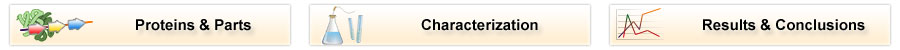# Team:TU Delft/Project/rbs-characterization/results

(Difference between revisions)
 Revision as of 14:13, 22 October 2010 (view source)← Older edit Revision as of 14:13, 22 October 2010 (view source)Newer edit → Line 26: Line 26: - ==RBS Characterization Results== ==RBS Characterization Results==
+ The given relative strengths are displayed assuming B0034 as the unit (The standard as used by the partsregistry). The given relative strengths are displayed assuming B0034 as the unit (The standard as used by the partsregistry). B0032 is defined to have a strength of 0.3. Therefore in the plot below, the mean value of B0032 is fixed on 0.3. B0032 is defined to have a strength of 0.3. Therefore in the plot below, the mean value of B0032 is fixed on 0.3. - [[Image:TUDelft2010_Rbs_strength_boxplot.png]] [[Image:TUDelft2010_Rbs_strength_boxplot.png]]

## RBS Characterization ResultsThe given relative strengths are displayed assuming B0034 as the unit (The standard as used by the partsregistry). B0032 is defined to have a strength of 0.3. Therefore in the plot below, the mean value of B0032 is fixed on 0.3.

Compared to the B0032, the tested Anderson RBS'es turned out to be surprisingly weak. All 5 of them resulted in less expression than the reference expression.

 RBS Mean strength Standard deviation J61100 0.026560 0.005126 J61117 0.123819 0.024725 J61101 0.081210 0.013402 J61107 0.019763 0.003963 J61127 0.069657 0.007422 B0032 0.300000 0.028316